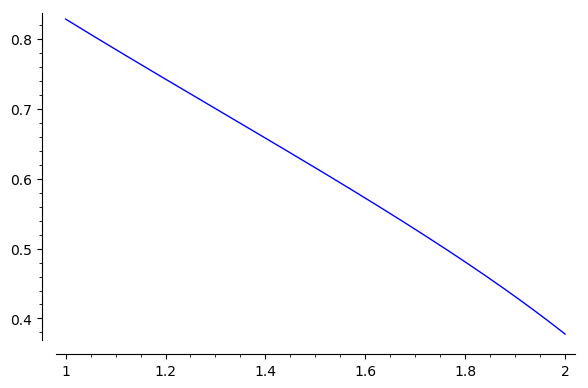Ask Your Question

# Revision history [back]

A different approach that works even in a Python 2 based Sage:

sage: var("y,z");
sage: cauchy(z) = y.subs(solve(z*y^3 + y^2 - 2*z*y + 2, y))
sage: F(z) = arctan2(imag(cauchy(z)),real(cauchy(z)))
sage: numerical_integral(F, 1, 2)
(0.6116545934235456, 6.790730127365591e-15)
sage: plot(F(z),(z,1,2))The plot is really slow. It may take more than 20 seconds. See this SageMath cell.